Computer Science

# Decomposition

We are going to spend some more time working on problems that require `decomposition`, which is the process of breaking a larger problem into smaller pieces.

Part of decomposition involves `abstraction`, meaning defining functions that can perform a particular job. When we define a function, we want to identify the following:

• `purpose` — the purpose of the function
• `boundary conditions` — what is happening before the function is called and after a function is called
• `interface` — the way that your program calls the function — what parameters it gives it (if any)

## Treasure

To practice these concepts, let’s examine the following problem. Bit needs to find the treasure! Here is the map: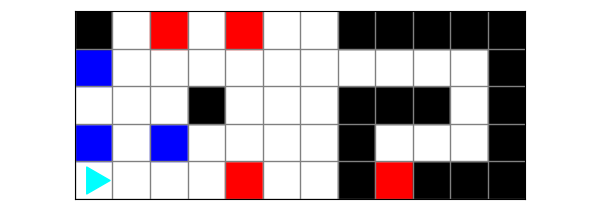To reach the cave, Bit should:

• Turn right on blue squares.
• Turn left on red squares.

Once in the cave, Bit should navigate through the passage to the treasure, turning left or right as needed to reach the red square.

### Planning

Work with a friend to draw this out and think about what steps you would use to solve it.Notice how there are two steps, and each step uses an event stream pattern.

### Getting started

The starter code is in the zip file above, in `treasure.py`:

``````from byubit import Bit

@Bit.worlds('treasure')
def go(bit):
# Write code here
pass

if __name__ == '__main__':
go(Bit.new_bit)``````

We can fill in the `run()` function with our basic plan:

``````def go(bit):
get_to_cave(bit)
find_treasure(bit)``````

Be sure to define these functions with `pass`:

``````def get_to_cave(bit):
pass

def find_treasure(bit):
pass``````

### Getting to the cave

(1) How does Bit get to the cave?

Bit should keep moving forward, turning left on red, right on blue.

(2) How does Bit know when it gets to the cave?

Bit reaches the cave when the left is not clear and the right is not clear.

Now that these are clearly defined, we can write several functions to get to the cave.

First, we will write a function to follow the turn instructions:

``````def follow_turn_instructions(bit):
""" Follow the rules for turning while navigating to the cave.
Turn left on red, right on blue.
"""
if bit.is_red():
bit.left()
elif bit.is_blue():
bit.right()``````

This is pretty simple! It just encodes the instructions we were given to follow the directions to the cave.

Next, we will write a function to know when to stop:

``````def in_cave(bit):
""" Return true if in the cave, otherwise false. """
return not bit.left_clear() and not bit.right_clear()``````

It can help to have a function like this because then our code that goes to the cave can just say `while not in_cave(bit)`.

Finally, we can write the `get_to_cave()` function:

``````def get_to_cave(bit):
"""
Bit ends just inside the cave (black on left and right)
To get there, Bit must turn right on blue and left on red.
"""
bit.paint('green')
while not in_cave(bit):
bit.move()
follow_turn_instructions(bit)
bit.paint('green')``````

Notice how simple this code is and how easy it is to read! This is the beauty of writing some helper functions.

This code follows the event stream pattern. See the definition of this pattern at the top of the page. In this pattern, we have a while loop, and then inside the while loop use `if` to make choices.

Finally, do be sure to paint after Bit follows the turning instructions! Otherwise, Bit will paint the red and blue squares green before using them to turn.

Let’s run the code to see how this works:Be sure to step through the code using the buttons so you can see how this works.

### Finding the treasure

Now we can write the `finding_the_treasure()` function.

(1) How does Bit get to the treasure?

Go forward, and if the front is blocked, turn left if it is clear on the left, or right if it is clear on the right.

(2) When does Bit know it has reached the treasure?

When it is on a red square.

Ok, let’s write a function to handle the first part:

``````def turn_to_clear(bit):
"""If left is clear, turn left, otherwise turn right."""
if bit.left_clear():
bit.left()
else:
bit.right()``````

This function turns Bit toward whichever direction is clear.

Now we can write a function for finding the treasure:

``````def find_treasure(bit):
"""Bit ends at the treasure (red square). If the front is blocked, turn in the direction that is clear."""
while not bit.is_red():
if not bit.front_clear():
turn_to_clear(bit)
bit.paint('green')
bit.move()

bit.paint('green')``````

Bit keeps moving as long as it is not on a red square. It turns as needed, and otherwise keeps moving and painting.\

How does this work?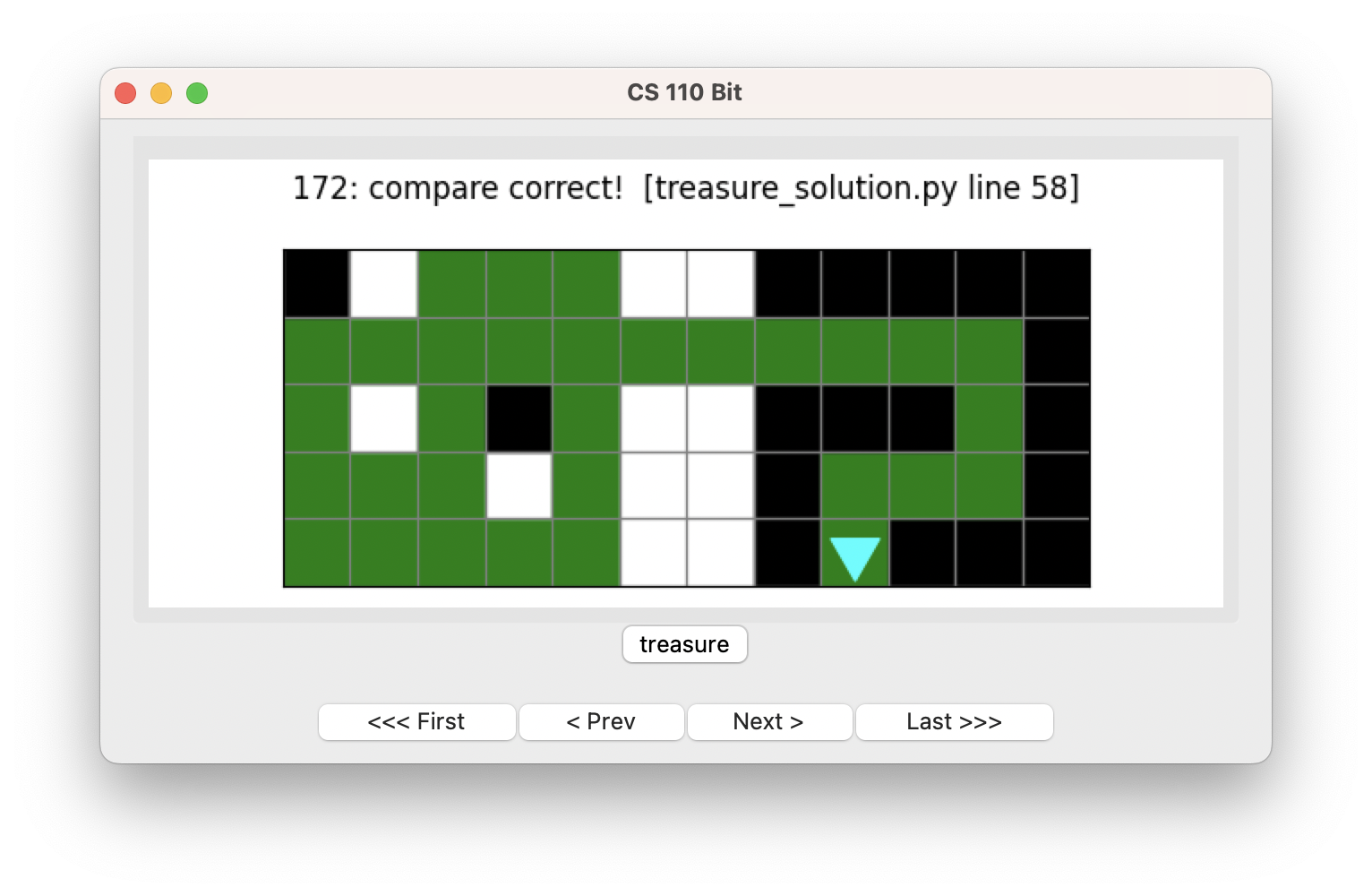Good job!

## Hiking

Let’s do one more problem. In this problem, Bit wants to hike over a mountain: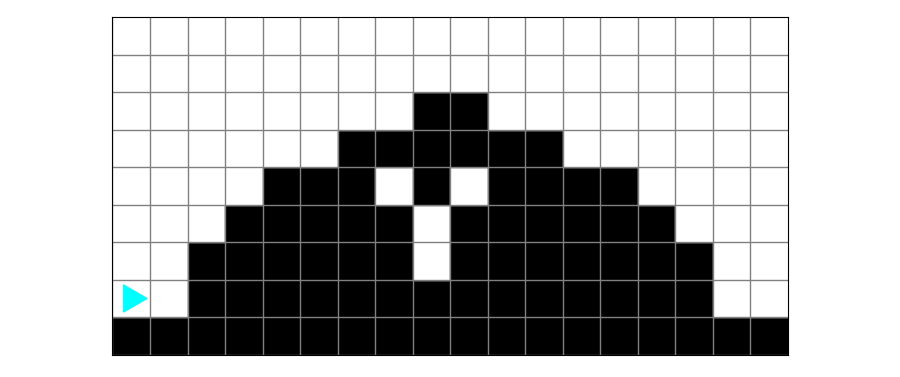Mountains can have different shapes: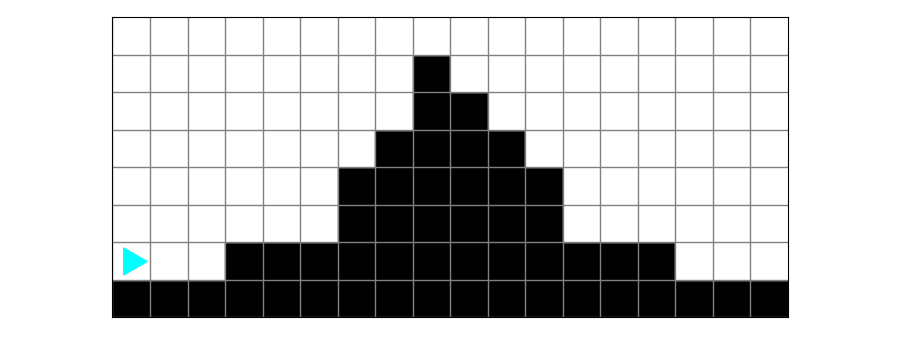Part of the decomposition process is understanding what the different examples have in commmon. We will continue to focus on the `event stream` pattern as a way of decomposing these problems.

When Bit hikes over a mountain, it plants a tree over every place where there is ground underneath it: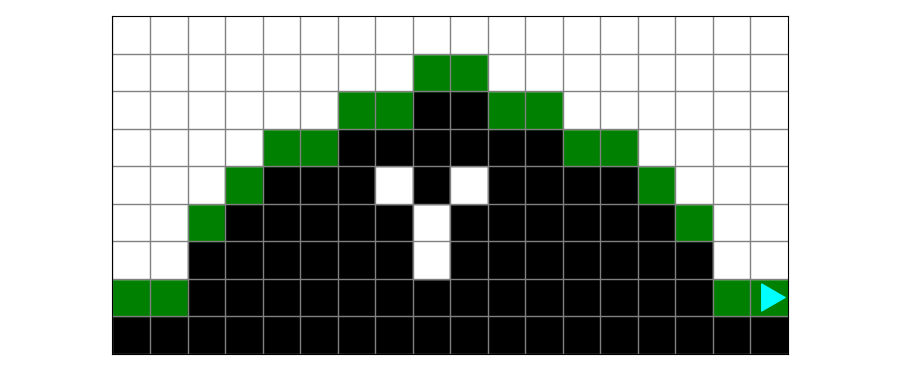### Planning

This is a tricky problem! Work with a friend to draw this out and think about what steps you would use to solve it.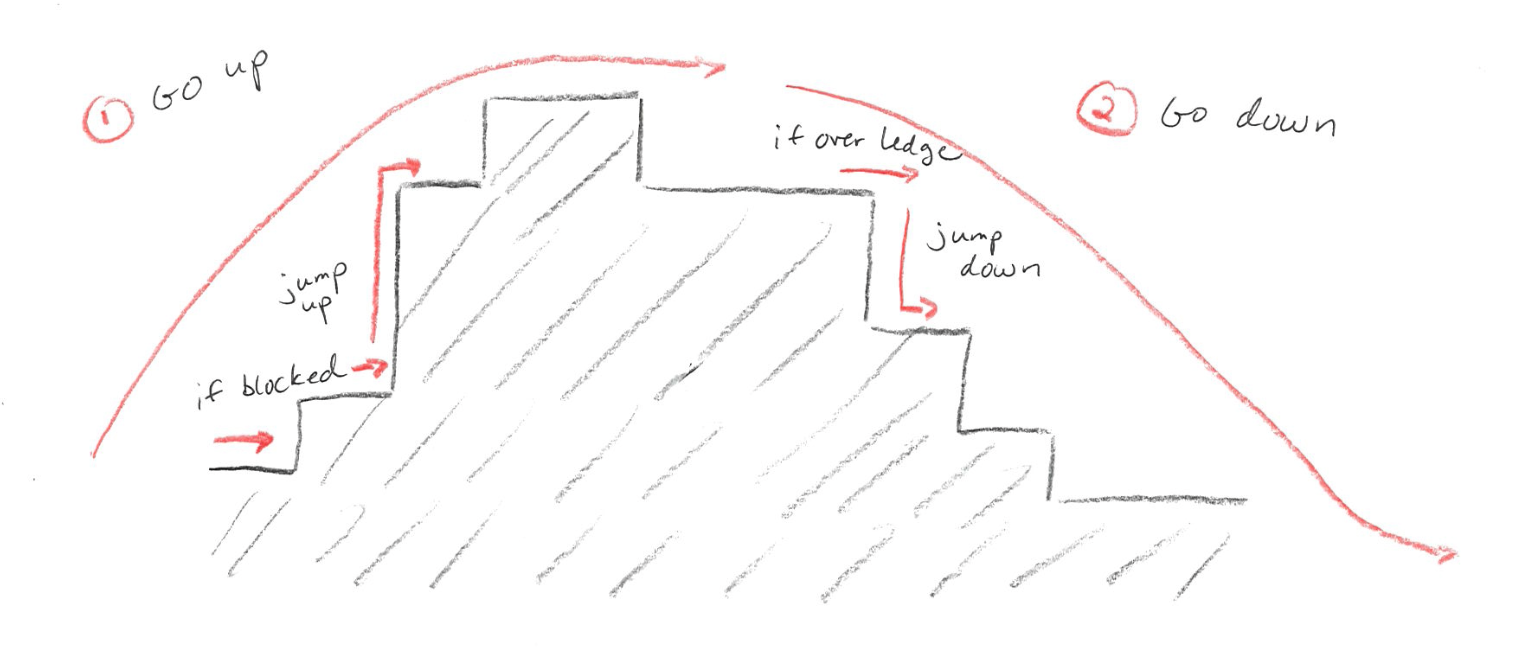• break the problem into going up and going down
• to go up, move forward as long as the right is clear
• if the front is blocked, jump up the ledge
• to go down, move forward as long as the front is clear
• if the right is clear, jump down the ledge

The key to this problem is to recognize it is hard to have a single event stream that covers the entire problem. When you are going up, you need to watch for when the front is blocked and “jump up” a ledge. But when you are going down, you need to watch for when the right is empty and “jump down” a ledge.

### Getting started

The starter code is in the zip file above, in `hike.py`:

``````from byubit import Bit

@Bit.worlds('y-mountain', 'mountain')
def run(bit):
# Write code here
pass

if __name__ == '__main__':
run(Bit.new_bit)``````

We can fill in the `run()` function with our basic plan:

``````def run(bit):
go_up(bit)
go_down(bit)``````

Be sure to define these functions with `pass`:

``````def go_up(bit):
pass

def go_down(bit):
pass``````

### Going up

Let’s start by going up. In `run()` we can call a function to go up as if it exists:

``````def run(bit):
go_up(bit)``````

Then we can write the `go_up()` function:

``````def go_up(bit):
""" Get Bit to the top of the mountain.

Bit ends at the top of the mountain facing right,
with an empty square to his right.

Bit paints green all the squares immediately above a black square.
"""
while not bit.right_clear():
if not bit.front_clear():
jump_up(bit)
bit.move()``````

The key to this function is getting the stopping condition right. When are we at the top of the mountain? When we reach a ledge that goes down, so it is clear on the right. This means we can use `while not bit.right_clear()` as our stopping condition.

Another important thing is to have a separate function for `jump_up()` whenever we reach a ledge and need to go up. We don’t know how high this ledge is and how many steps this will take, so it works well to write a separate function for `jump_up()`:

``````def jump_up(bit):
""" Jump up the ledge.
Start facing the wall at the bottom of the ledge.
End at the top, facing right, with an empty square underneath
(Bit is not "on" the ledge yet)
"""
bit.left()
while not bit.right_clear():
bit.move()

bit.right()``````

Notice how this needs its own logic — turn left, then move as long as the right is clear, then turn right. You could put this in the `go_up()` function, but if you find yourself with nested while loops, that is a good reason to make a separate function.

Notice also that when Bit goes up a ledge it has the right clear. This is the stopping condition for going up the mountain in `go_up()`! So this is another good reason to have a separate function for jumping up, so you don’t confuse the logic with knowing when you are at the top of the mountain.

Let’s run what we have so far: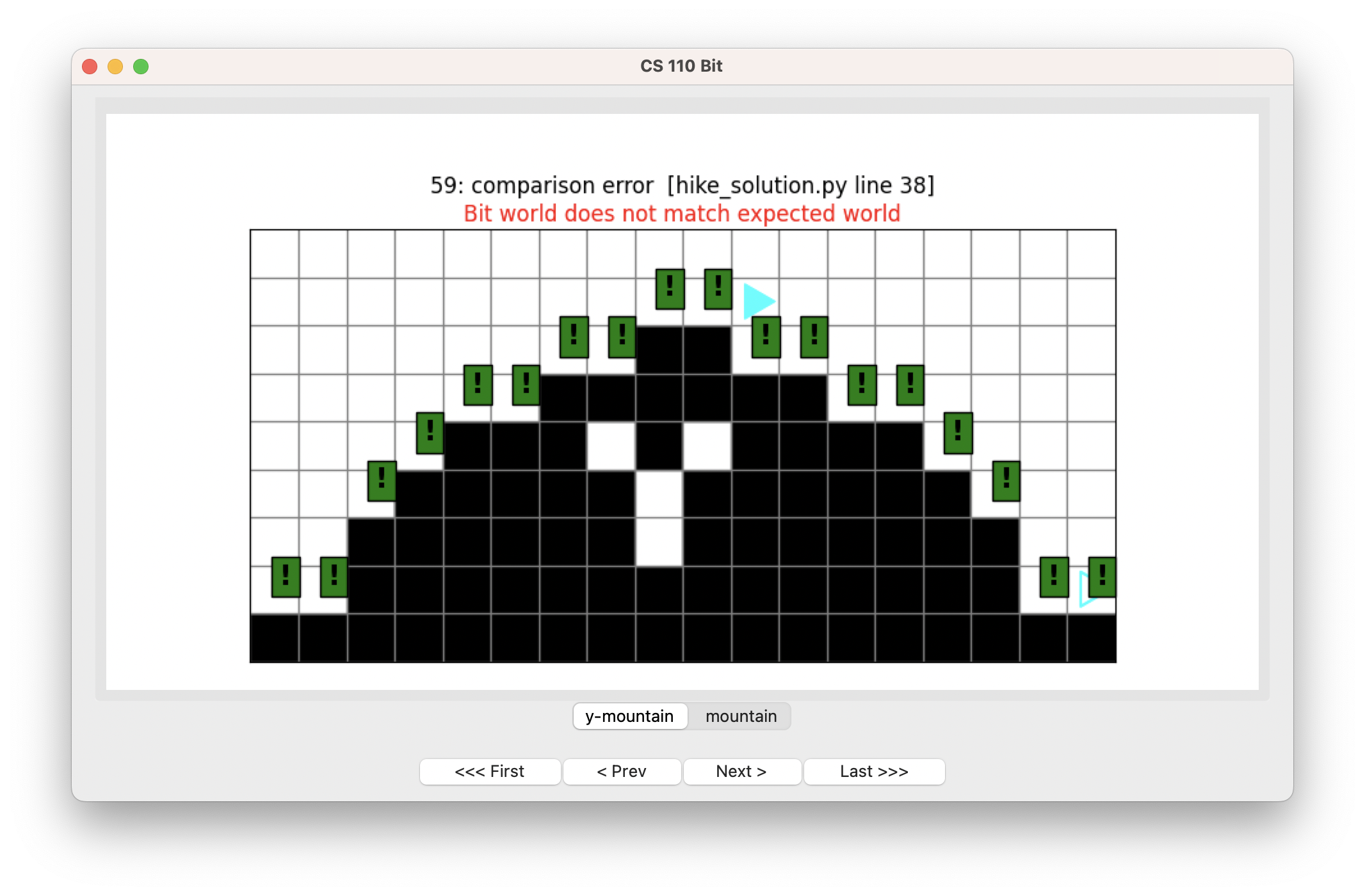OK, not bad! Bit has made it up the mountain to exactly the place we want it to be — right over the first ledge going down. But we haven’t planted any trees. Let’s do that next.

### Planting trees

To plant trees, we need a function:

``````def plant_a_tree(bit):
""" If the square below is black, plant a tree. """
if not bit.right_clear():
bit.paint('green')``````

This will plant a tree if there is ground underneath Bit — it is not clear. Having a separate function is wise because we will also need it when Bit goes down.

We can call this function inside the `go_up()` function:

``````def go_up(bit):
""" Get Bit to the top of the mountain.

Bit ends at the top of the mountain facing right,
with an empty square to his right.

Bit paints green all the squares immediately above a black square.
"""
plant_a_tree(bit)
while not bit.right_clear():
if not bit.front_clear():
jump_up(bit)
bit.move()
plant_a_tree(bit)``````

Note that we don’t need to call it inside `jump_up()` because there is never ground underneath Bit when it jumps up.

Let’s try that: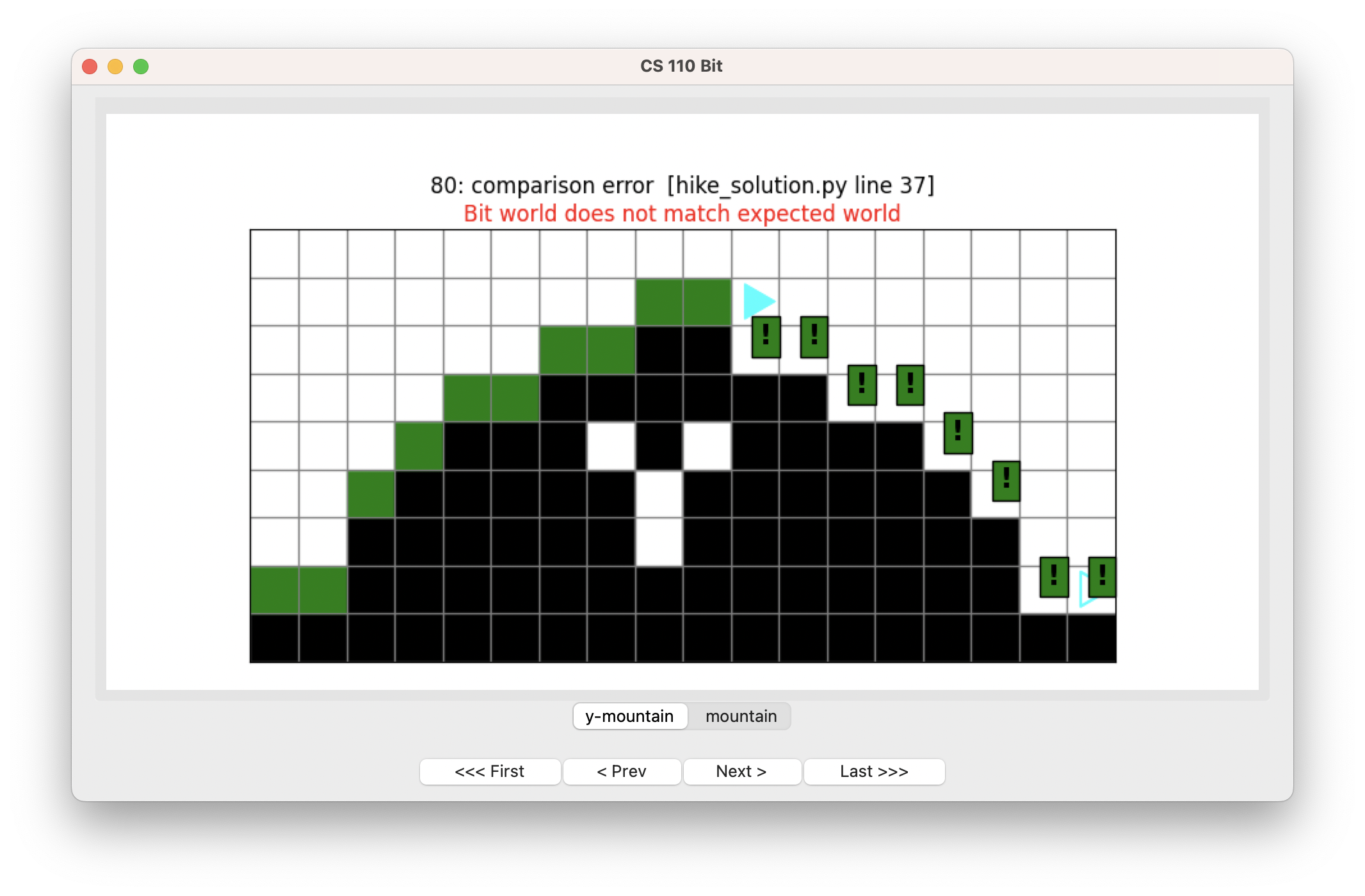Nice!

### Going down

Now we can write `go_down()`:

``````def go_down(bit):
""" Bit starts at the top of the mountain (facing right,
empty space beneath) and descends to the right,
ending in the corner.
"""
while bit.front_clear():
if bit.right_clear():
jump_down(bit)
bit.move()``````

We want to do something similar as going up, except Bit keeps moving while the front is clear. Then, any time it is over an edge, we tell Bit to jump down.

Jumping down is similar to jumping up, but Bit moves while the front is clear.

``````def jump_down(bit):
""" Bit starts facing right with empty beneath,
and ends facing right with black beneath.
"""
bit.right()
while bit.front_clear():
bit.move()

bit.left()``````

Let’s see how this works: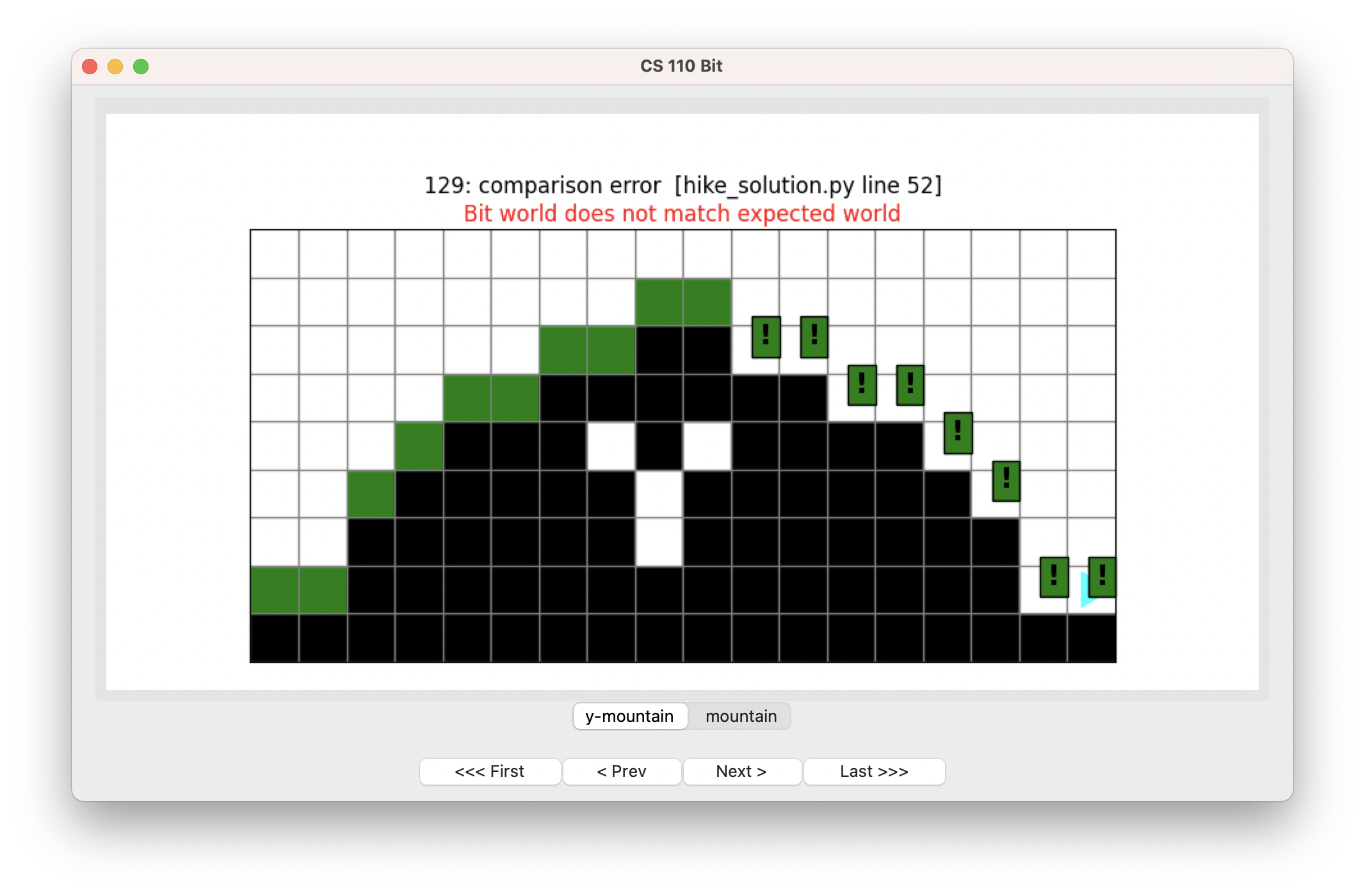OK, we just need to plant trees again!

### Planting trees (again)

Where should we call `plant_a_tree()`? Let’s try what we did when going up, planting a tree after Bit moves:

``````def go_down(bit):
""" Bit starts at the top of the mountain (facing right,
empty space beneath) and descends to the right,
ending in the corner.
"""
while bit.front_clear():
if bit.right_clear():
jump_down(bit)
bit.move()
plant_a_tree(bit)``````

Let’s run this: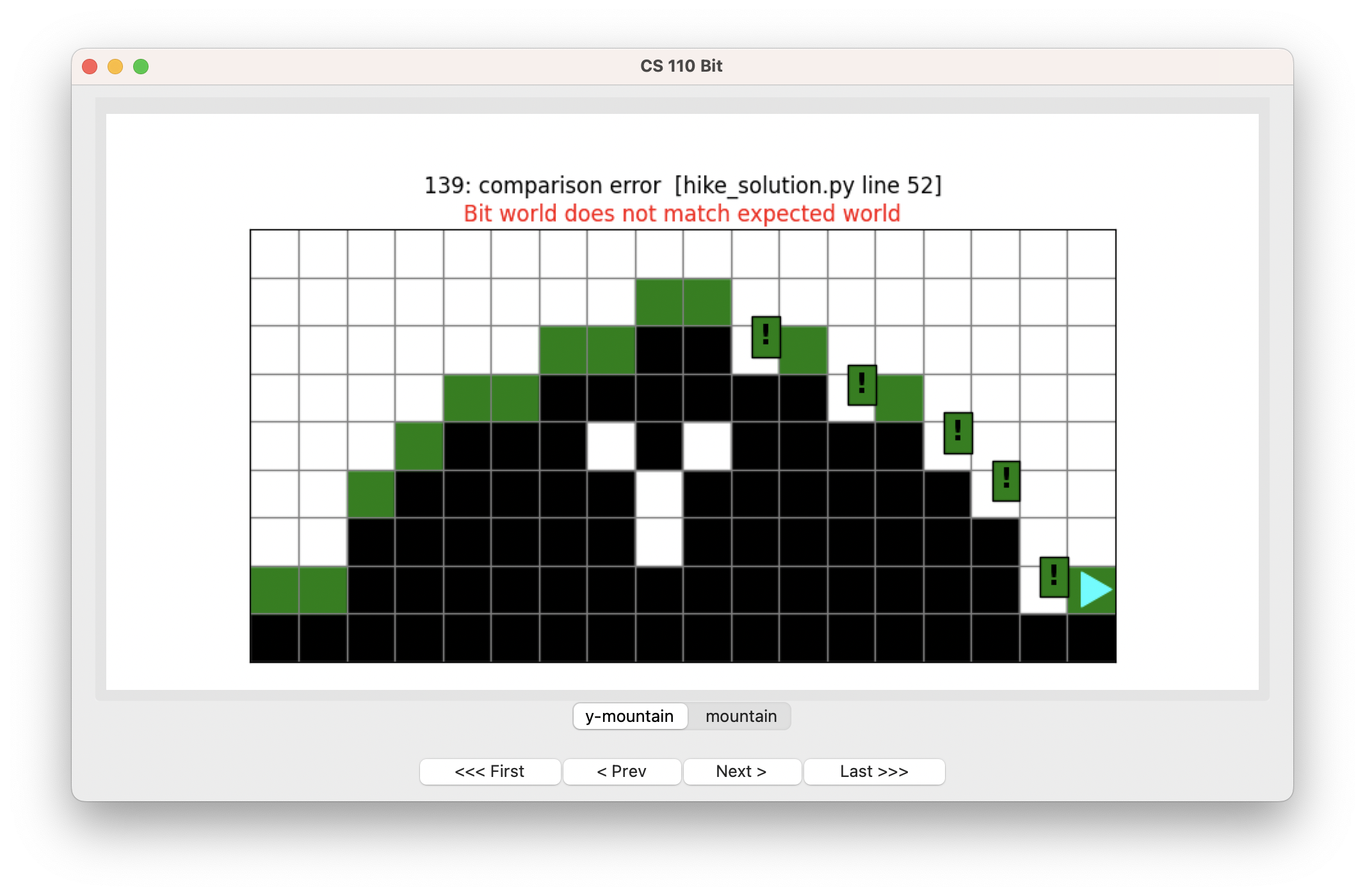Oops! Bit is planting trees but skipping some spots. Use the buttons to see if you can figure out why.When Bit comes down off a ledge, it moves and then paints, so it is missing trees right at its landing spot. We can fix this by planting before moving:

``````def go_down(bit):
""" Bit starts at the top of the mountain (facing right,
empty space beneath) and descends to the right,
ending in the corner.
"""
while bit.front_clear():
if bit.right_clear():
jump_down(bit)

plant_a_tree(bit)
bit.move()

plant_a_tree(bit)``````

That means we also need a final `plant_a_tree()` at the end to get the last square.

If we run this: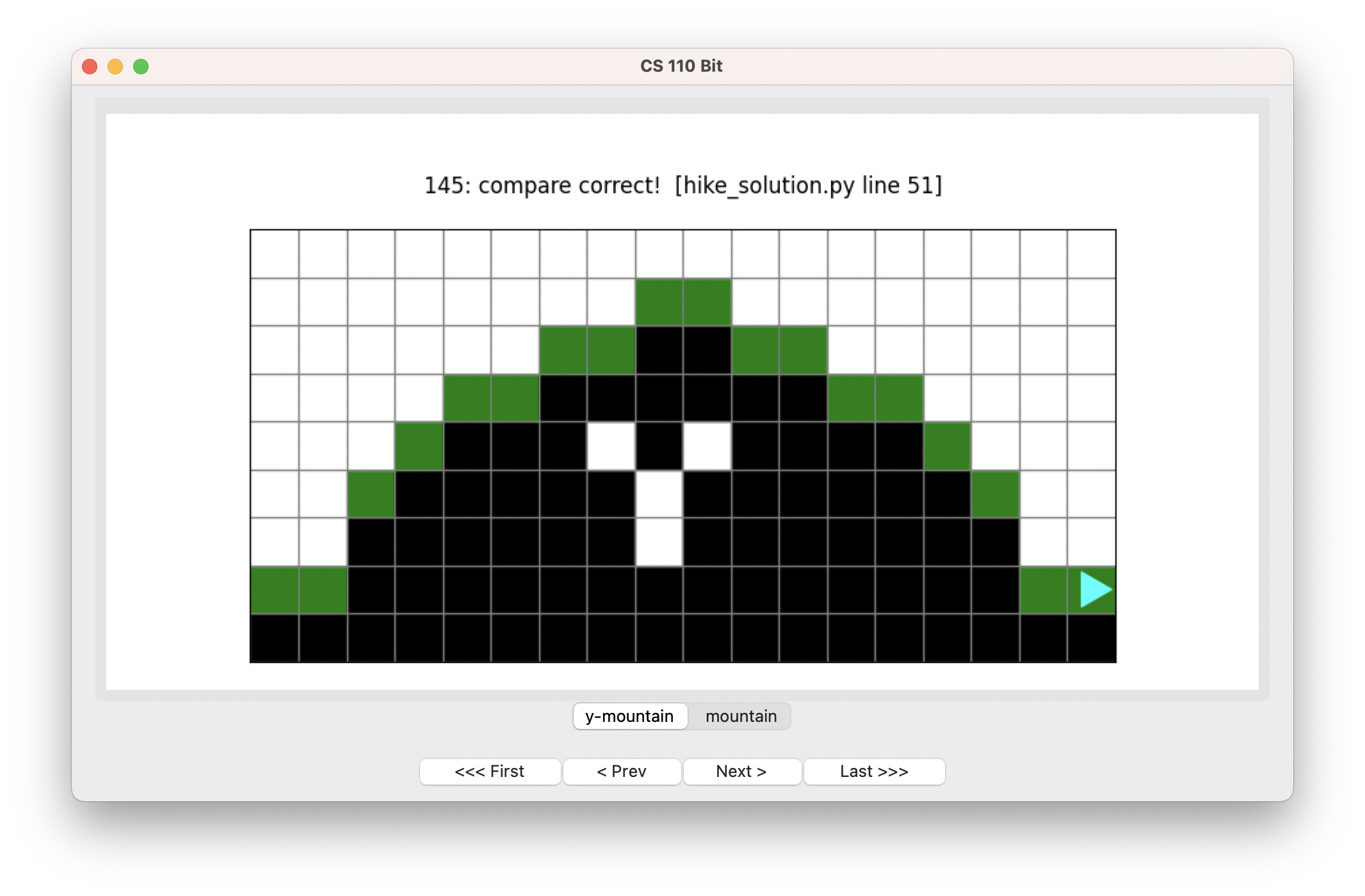Hooray! Problem solved! And it works for the other world too: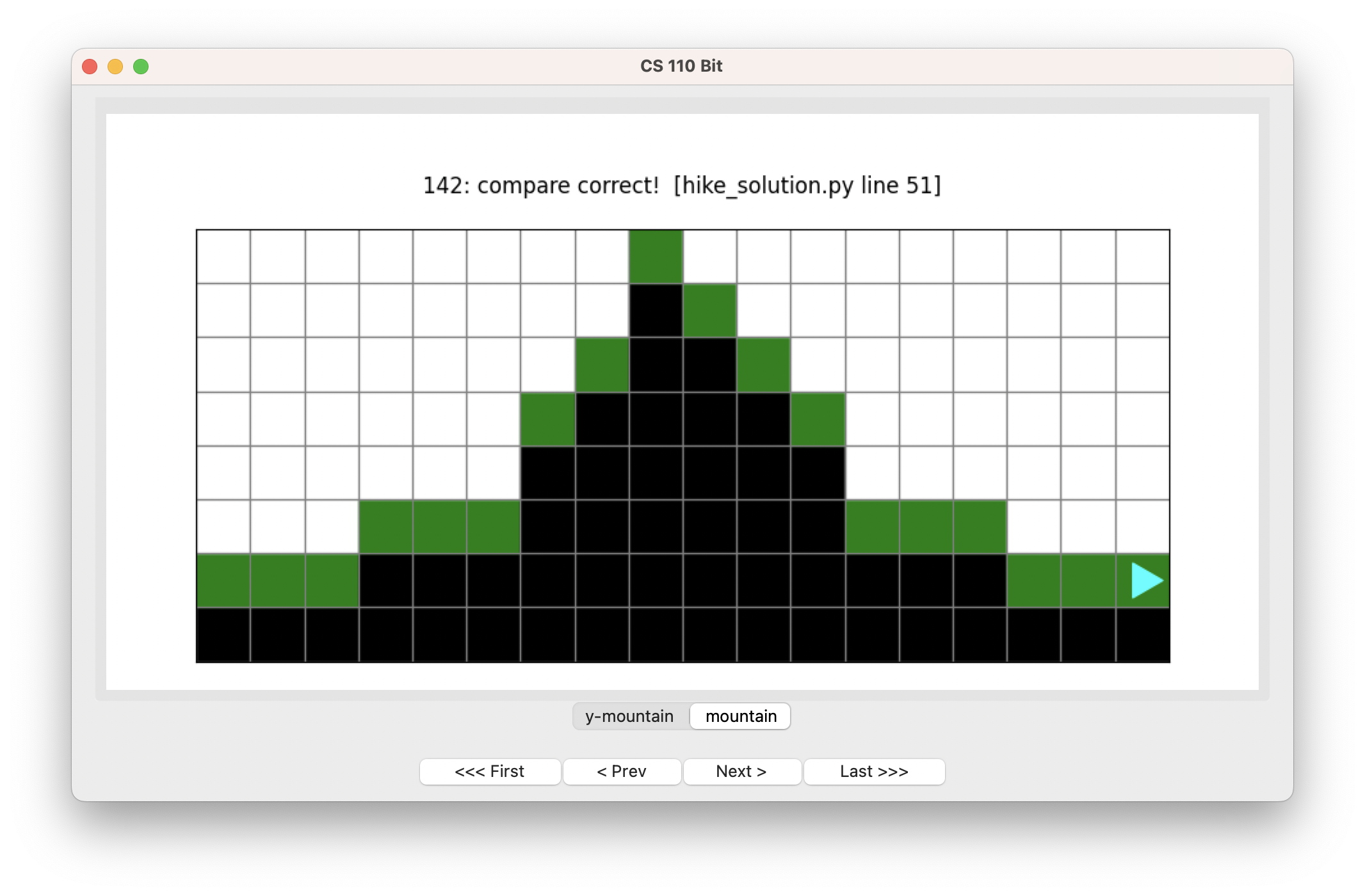## Do yourself a favor

Try to write code like this! It will bless your life! :-)

1. Plan a problem out in advance, with a drawing and/or a flow chart.

2. Decompose the problem into multiple functions, each one doing one thing.

3. Work through each function carefully, and one or a few at a time. Test as you go.

4. Write clear comments to document each function.